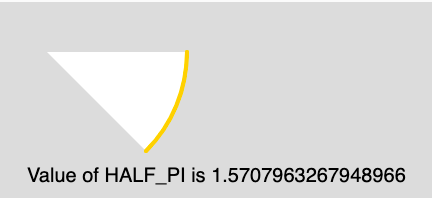GeeksforGeeks App
Open AppBrowser
Continue

## Related Articles

The HALF_PI is a mathematical constant and its value is 1.57079632679489661923. The HALF_PI is the half ratio of the circumference of a circle to its diameter.

Syntax:

`HALF_PI`

Below program illustrates the usage of HALF_PI in p5.js:

Example:

 `function` `setup() {`` ` `    ``// Create Canvas of size 300*200``    ``createCanvas(300, 200);``}`` ` `function` `draw() {``     ` `    ``// Set the background Color``    ``background(220);``     ` `    ``// Set the stroke color``    ``stroke(255, 204, 0);``     ` `    ``// Set the stroke weight``    ``strokeWeight(4);``     ` `    ``// Use of constant HALF_PI``    ``arc(50, 50, 280, 280, 0, HALF_PI/2); ``     ` `    ``noStroke();``     ` `    ``// Set the font size``    ``textSize(20);``     ` `    ``// Display result``    ``text(``"Value of HALF_PI is "``            ``+ HALF_PI, 30, 180);``}`

Output:Reference: https://p5js.org/reference/#/p5/HALF_PI

My Personal Notes arrow_drop_up
Related Tutorials